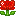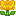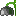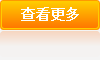我要留言

 客户姓名： 留言主题： 客户评价：好评中评差评 留言内容： 告诉演讲网老师们一个好消息，昨天竞聘演讲成绩出来了，我得了****分，一举挫败了大家一致看好的竞争对手。我知道，之所以能够成功，除了平时工作比较踏实外，一份精彩的演讲稿帮了我大忙。在这留个言，报个喜，谢谢演讲网的专家！下次，有稿子，我还找你们写！<% sql_="select * from J_liuyan where shenhe=1 order by addtime desc, id desc" set rs_=conn.execute(sql_) for i=1 to 10 if rs_.eof then exit for %> <% rs_.movenext next %>
 客户： <%=rs_("username")%> <% if rs_("classid")=1 then%>好评<% end if %> <% if rs_("classid")=2 then%>中评<% end if %> <% if rs_("classid")=3 then%>差评<% end if %> ','','width=500,height=400')"><%=rs_("title")%>